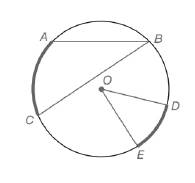Chapter 6.1, Problem 4EElementary Geometry For College St...

7th Edition
Alexander + 2 others
ISBN: 9781337614085

Solutions

Chapter
SectionElementary Geometry For College St...

7th Edition
Alexander + 2 others
ISBN: 9781337614085
Textbook Problem

For Exercises 1 to 8, use the figure provided.Exercises 1-8If m A C ⌢ = 56.4 ∘ ,  find m ∠ B .

To determine

To calculate:

The mB, if mAC=56.4 by using the provided figure.

Explanation

Given:

The provided figure is

Theorem used:

The measure of an inscribed angle of a circle is one-half the measure of its intercepted arc.

Calculation:

To find: mB.

Given that, mAC=56.4.

Therefore, mB is the one-half the measure of its intercepted arc.

Here the intercepted arc is AC.

Still sussing out bartleby?

Check out a sample textbook solution.

See a sample solution

The Solution to Your Study Problems

Bartleby provides explanations to thousands of textbook problems written by our experts, many with advanced degrees!

Get Started

Find more solutions based on key concepts